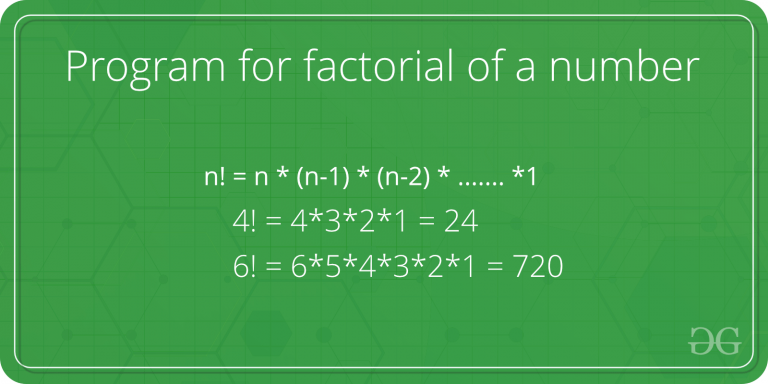# C Program for factorial of a number

Factorial of a non-negative integer is multiplication of all integers smaller than or equal to n. For example factorial of 6 is 6*5*4*3*2*1 which is 720.Recursive:

 `// C program to find factorial of given number ` ` `  `#include ` ` `  `// Function to find factorial of given number ` `unsigned ``int` `factorial(unsigned ``int` `n) ` `{ ` `    ``if` `(n == 0) ` `        ``return` `1; ` `    ``return` `n * factorial(n - 1); ` `} ` ` `  `// Driver code ` `int` `main() ` `{ ` `    ``int` `num = 5; ` `    ``printf``(``"Factorial of %d is %d"``, ` `           ``num, factorial(num)); ` `    ``return` `0; ` `} `

Output:

```Factorial of 5 is 120
```

Iterative :

 `#include ` ` `  `// Function to find factorial of given number ` `unsigned ``int` `factorial(unsigned ``int` `n) ` `{ ` `    ``int` `res = 1, i; ` `    ``for` `(i = 2; i <= n; i++) ` `        ``res *= i; ` `    ``return` `res; ` `} ` ` `  `// Driver code ` `int` `main() ` `{ ` `    ``int` `num = 5; ` `    ``printf``(``"Factorial of %d is %d"``, ` `           ``num, factorial(num)); ` `    ``return` `0; ` `} `

Output:

```Factorial of 5 is 120
```

One line Solution (Using Ternary operator):

 `// C++ program to find factorial of given number ` ` `  `#include ` ` `  `int` `factorial(``int` `n) ` `{ ` `    ``// single line to find factorial ` `    ``return` `(n == 1 || n == 0) ` `               ``? 1 ` `               ``: n * factorial(n - 1); ` `} ` ` `  `// Driver code ` `int` `main() ` `{ ` `    ``int` `num = 5; ` `    ``printf``(``"Factorial of %d is %d"``, ` `           ``num, factorial(num)); ` `    ``return` `0; ` `} ` `// This code is contributed by  Rithika palaniswamy. `

Output:

```Factorial of 5 is 120
```

Using tgamma() method:

Example:

```Input: n = 4
Output: 24
```

Syntax:

```tgamma(n+1)=n!
It works upto 20! because c can't store large value
```

Implementation: use math.h header file for this

 `#include ` `#include ` ` `  `int` `main() ` `{ ` ` `  `    ``// use long long int ` `    ``// for larger values of n ` `    ``int` `n = 4; ` ` `  `    ``// tgamma(n+1)=n! ` `    ``n = tgamma(n + 1); ` ` `  `    ``printf``(``"%d"``, n); ` `    ``return` `0; ` `    ``// This code is contributed by Soumyadip Basak ` `} `

Output:

```24
```

The above solutions cause overflow for large numbers. Please refer factorial of large number for a solution that works for large numbers.

Please refer complete article on Program for factorial of a number for more details!

My Personal Notes arrow_drop_up